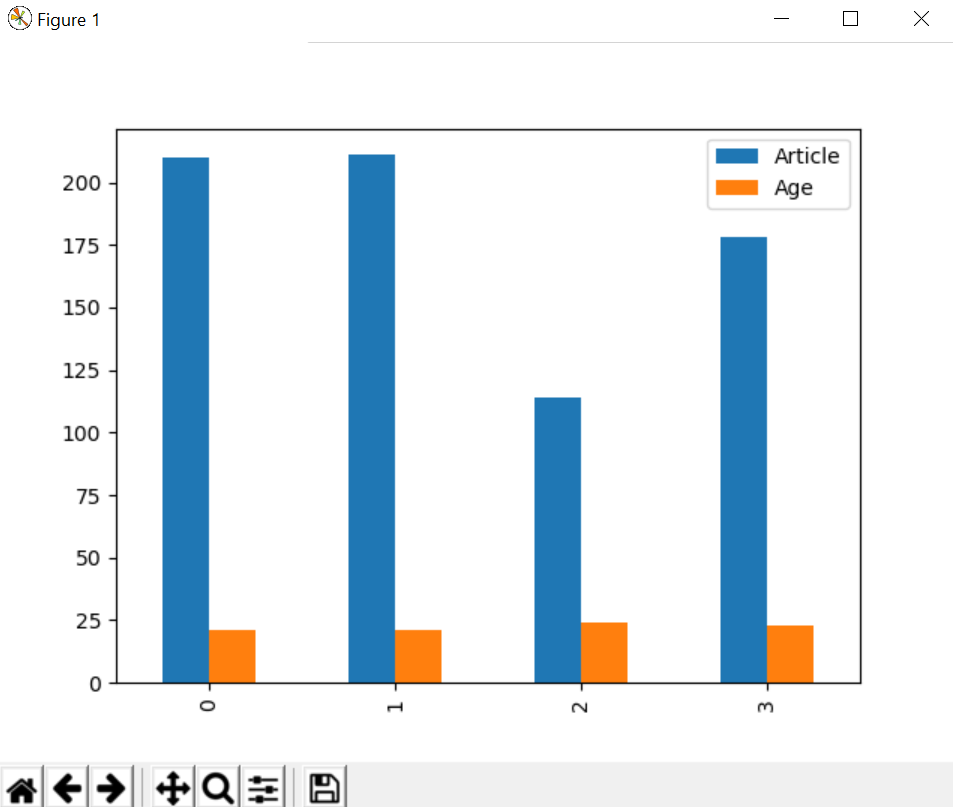Creating a dataframe from Pandas series

Series is a type of list in pandas which can take integer values, string values, double values and more. But in Pandas Series we return an object in the form of list, having index starting from 0 to n, Where n is the length of values in series.

Later in this article, we will discuss dataframes in pandas, but we first need to understand the main difference between Series and Dataframe. Series can only contain single list with index, whereas dataframe can be made of more than one series or we can say that a dataframe is a collection of series that can be used to analyse the data.

Code #1: Creating a simple Series

 import pandas as pd import matplotlib.pyplot as plt    author = ['Jitender', 'Purnima', 'Arpit', 'Jyoti']    auth_series = pd.Series(author) print(auth_series)

Output:

0    Jitender
1     Purnima
2       Arpit
3       Jyoti
dtype: object

Let’s check type of Series:

 import pandas as pd import matplotlib.pyplot as plt    author = ['Jitender', 'Purnima', 'Arpit', 'Jyoti']    auth_series = pd.Series(author) print(type(auth_series))

Output:

<class 'pandas.core.series.Series'>

Code #2: Creating Dataframe from Series

 import pandas as pd import matplotlib.pyplot as plt    author = ['Jitender', 'Purnima', 'Arpit', 'Jyoti'] article = [210, 211, 114, 178]    auth_series = pd.Series(author) article_series = pd.Series(article)    frame = { 'Author': auth_series, 'Article': article_series }    result = pd.DataFrame(frame)    print(result)

Output:

Author  Article
0  Jitender      210
1   Purnima      211
2     Arpit      114
3     Jyoti      178

Explanation:
We are combining two series Author and Article published. Create a dictionary so that we can combine the metadata for series. Metadata is the data of data that can define the series of values. Pass this dictionary to pandas DataFrame and finally you can see the result as combination of two series i.e for author and number of articles.

Code #3: How to add series externally in dataframe

 import pandas as pd import matplotlib.pyplot as plt    author = ['Jitender', 'Purnima', 'Arpit', 'Jyoti'] article = [210, 211, 114, 178]    auth_series = pd.Series(author) article_series = pd.Series(article)    frame = { 'Author': auth_series, 'Article': article_series }    result = pd.DataFrame(frame) age = [21, 21, 24, 23]    result['Age'] = pd.Series(age)    print(result)

Output:

Author  Article  Age
0  Jitender      210   21
1   Purnima      211   21
2     Arpit      114   24
3     Jyoti      178   23

Explanation:
We have added one more series externally named as age of the authors, then directly added this series in the pandas dataframe. Remember one thing if any value is missing then by default it will be converted into NaN value i.e null by default.

Code #4: Missing value in dataframe

 import pandas as pd import matplotlib.pyplot as plt    author = ['Jitender', 'Purnima', 'Arpit', 'Jyoti'] article = [210, 211, 114, 178]    auth_series = pd.Series(author) article_series = pd.Series(article)    frame = { 'Author': auth_series, 'Article': article_series }    result = pd.DataFrame(frame) age = [21, 21, 23]    result['Age'] = pd.Series(age)    print(result)

Output:

Author  Article   Age
0  Jitender      210  21.0
1   Purnima      211  21.0
2     Arpit      114  23.0
3     Jyoti      178   NaN

Code #5: Data Plot on graph

Using plot.bar() we have created a bar graph.

 import pandas as pd import matplotlib.pyplot as plt    author = ['Jitender', 'Purnima', 'Arpit', 'Jyoti'] article = [210, 211, 114, 178]    auth_series = pd.Series(author) article_series = pd.Series(article)    frame = { 'Author': auth_series, 'Article': article_series }    result = pd.DataFrame(frame) age = [21, 21, 24, 23]    result['Age'] = pd.Series(age)    result.plot.bar() plt.show()

Output:My Personal Notes arrow_drop_upCheck out this Author's contributed articles.

If you like GeeksforGeeks and would like to contribute, you can also write an article using contribute.geeksforgeeks.org or mail your article to contribute@geeksforgeeks.org. See your article appearing on the GeeksforGeeks main page and help other Geeks.

Please Improve this article if you find anything incorrect by clicking on the "Improve Article" button below.

Article Tags :

1

Please write to us at contribute@geeksforgeeks.org to report any issue with the above content.